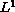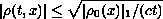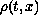Electron. J. Diff. Eqns., Vol. 2003(2003), No. 23, pp. 1-11.

### L^1 singular limit for relaxation and viscosity approximations of extended traffic flow models Christian Klingenberg, Yun-guang Lu, & Hui-jiang Zhao

Abstract:
This paper considers the Cauchy problem for an extended traffic flow model with-bounded initial data. A solution of the corresponding equilibrium equation with-bounded initial data is given by the limit of solutions of viscous approximations of the original system as the dissipation parametertends to zero more slowly than the response time. The proof of convergence is obtained by applying the Young measure to solutions introduced by DiPerna and, based on the estimatederived from one of Lax's results and Diller's idea, the limit functionis shown to be a-entropy week solution. A direct byproduct is that we can get the existence of-entropy solutions for the Cauchy problem of the scalar conservation law with-bounded initial data without any restriction on the growth exponent of the flux function provided that the flux function is strictly convex. Our result shows that, unlike the weak solutions of the incompressible fluid flow equations studied by DiPerna and Majda in , for convex scalar conservation laws with-bounded initial data, the concentration phenomenon will never occur in its global entropy solutions.

Submitted October 25, 2002. Published March 7, 2003.
Math Subject Classifications: 35B40, 35L65.
Key Words: Singular limit, traffic flow model, relaxation and viscosity approximation.

Show me the PDF file (221K), TEX file, and other files for this article.Christian Klingenberg Department of Mathematicas, Wurzburg University Wurzburg, 97074, Germany e-mail: klingen@mathematik.uni-wuerzburg.de Yun-guang Lu Department of Mathematics University of Science and Technology of China, Hefei, China and Departamento de Matematicas Universidad Nacional de Colombia, Bogota, Colombia e-mail: yglu@matematicas.unal.edu.co Hui-jiang Zhao Institute of Physics and Mathematicas Chinese Academy of Sciences, Wuhan, China e-mail: hjzhao@wipm.whcnc.ac.cn